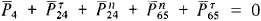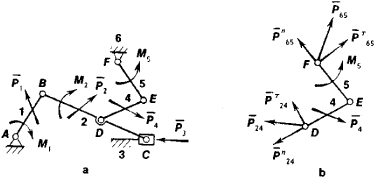# Dynamic Force Analysis of Mechanisms

The following article is from The Great Soviet Encyclopedia (1979). It might be outdated or ideologically biased.

## Dynamic Force Analysis of Mechanisms

a branch of the theory of machines and mechanisms. It uses calculation of forces to determine the reactions of the elements of kinematic pairs of a mechanism, assuming that the law of motion for the mechanism is known. The methods of dynamic force analysis are used for strength calculation in the design of new machines.

If inertial forces are added to all external forces acting on the members of a mechanism, then according to d’Alembert’s principle the mechanism as a whole and its individual parts can be taken to be in a state of equilibrium. Therefore, static equations can be used in determining the forces acting on a mechanism (the reactions). Systems of equations are written for parts of the mechanism—that is, for its members and for kinematic pairs. The number of unknown reactions equals the number of equations. In mechanics such systems are called statically determinable. The force calculation of a mechanism is made in sequence for the kinematic pairs, starting with the group farthest removed from the originating member of the mechanism. For example, a mechanism (Figure l,a) consists of the originating member (1) and kinematic pairs containing members (2)-(3) and (4)-(5). The members are acted upon by forces P1, P2, P3, and P4, including the inertial loads, and by the moments M1, M2, and M5. For a force calculation the group (4)-(5) of the mechanism is considered first (Figure l,b). The unknown reactions P24 and P̄65 which are resolved into their normal components n24 and τ65 and their tangential components T24 and T65 are substituted for the action of members (6) and (2) on the group. The tangential components are determined from the equation for the sum of moments with respect to point E for each of themembers (4) and (5). The normal components n24 and n65—and consequently the total reactions P24 and P65—are determined from the vector equilibrium equation of the group:To solve the vector equation a force polygon is constructed. The reaction P̄45 = —P̄54 is determined from the vector equation of force equilibrium on one of the members (4) or (5). The group (2)-(3) is considered next. In addition to the given forces, this group is acted upon by the reaction P̄42 = —P̄24 that was found. The reaction P61, as well as the balancing moment Mb, is found in considering the equilibrium of the originating member (1). The latter moment, applied to this member, is necessary to make valid the law of motion given for the originating member.Figure 1. Diagram of forces acting in a mechanism (a) and determination of the reactions in a member (b): (P1,), (P2), (P3), and (P4) acting forces; (M1), (M2), and (M5) moments of the forces; (P24) and (P25) unknown reactions

If forces of friction in kinematic pairs are taken into account, one more independent equation is added to the system of equations. After determining the reactions, the forces of friction in the pairs are computed and the calculation is repeated, taking forces of friction into account as external forces applied to the members—that is, more exact reactions are determined in the first approximation. The calculation may be repeated with given forces of friction taken into account. In practice the first approximation is usually satisfactory. In force computations for multimember spatial mechanisms the method and sequence of dynamic force analysis are preserved, but the solutions become more cumbersome.

I. I. ARTOBOLEVSKII and A. P. BESSONOV

The Great Soviet Encyclopedia, 3rd Edition (1970-1979). © 2010 The Gale Group, Inc. All rights reserved.
Site: Follow: Share:
Open / Close